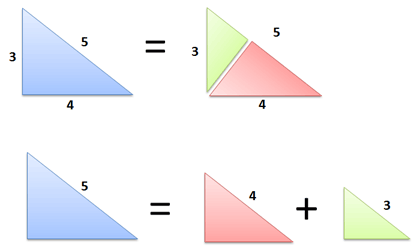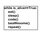# Great Uses Of Pythagoras TheoremIntuitive Look at The Pythagorean Theorem We can all agree the Pythagorean Theorem is true . But most proofs offer a mechanical understanding: re-arrange the shapes, and voila, the equation holds. But is it really clear, intuitively, that it must be a2 + b2 = c2 and not 2a2 + b2 = c2? No? Well, let's build some intuition.

There's one killer concept we need: Any right triangle can be split into two similar right triangles.

Cool, huh? Drawing a perpendicular line through the point splits a right triangle into two smaller ones. Geometry lovers, try the proof yourself: use angle-angle-angle similarity. Area (Big) = Area (Medium) + Area (Small) Makes sense, right? The smaller triangles were cut from the big one, so the areas must add up. And the kicker: because the triangles are similar, they have the same area equation.

Let's call the long side c (5), the middle side b (4), and the small side a (3). Our area equation for these triangles is:

Area = F * hypotenuse^2}

where F is some area factor (6/25 or .24 in this case; the exact number doesn't matter). Now let's play with the equation:

{Area (Big) = Area (Medium) + Area (Small)}

{F c^2 = F b^2 + F a^2}

Divide by F on both sides and you get:

{c^2 = b^2 + a^2}

Which is our famous theorem! You knew it was true, but now you know why:

A triangle can be split into two smaller, similar ones Since the areas must add up, the squared hypotenuses (which determine area) must add up as well. This takes a bit of time to see, but I hope the result is clear. How could the small triangles not add to the larger one?

Actually, it turns out the Pythagorean Theorem depends on the assumptions of Euclidean geometry and doesn't work on spheres or globes, for example. But we'll save that discussion for another time.

Useful Application: Try Any Shape We used triangles in our diagram, the simplest 2-D shape. But the line segment can belong to any shape. Take circles, for example:

Now what happens when we add them together?

You guessed it: Circle of radius 5 = Circle of radius 4 + Circle of radius 3.

Pretty wild, eh? We can multiply the Pythagorean Theorem by our area factor (pi, in this case) and come up with a relationship for any shape.

Remember, the line segment can be any portion of the shape. We could have picked the circle's radius, diameter, or circumference -- there would be a different area factor, but the 3-4-5 relationship would still hold.

So, whether you're adding up pizzas or Richard Nixon masks, the Pythagorean theorem helps you relate the areas of any similar shapes. Now that's something they didn't teach you in grade school.

Useful Application: Conservation of Squares The Pythagorean Theorem applies to any equation that has a square. The triangle-splitting means you can split any amount (c2) into two smaller amounts (a2 + b2) based on the sides of a right triangle. In reality, the "length" of a side can be distance, energy, work, time, or even people in a social network:

Social Networks.

Metcalfe's Law (if you believe it) says the value of a network is about n2 (the number of relationships). In terms of value,

Network of 50M = Network of 40M + Network of 30M. Pretty amazing -- the 2nd and 3rd networks have 70M people total, but they aren't a coherent whole. The network with 50 million people is as valuable as the others combined.

Computer Science

Some programs with n inputs take n2 time to run (bubble sort, for example). In terms of processing time

50 inputs = 40 inputs + 30 inputs Pretty interesting. 70 elements spread among two groups can be sorted as fast as 50 items in one group. (Yeah, there may be constant overhead/start up time, just work with me here).

Given this relationship, it makes sense to partition elements into separate groups and then sort the subgroups. Indeed, that's the approach used in quicksort, one of the best general-purpose sorting methods. The Pythagorean theorem helps show how sorting 50 combined elements can be as slow as sorting 30 and 40 separate ones.

Surface Area

The surface area of a sphere is 4 pi r2. So, in terms of surface area of spheres:

Area of radius 50 = area of radius 40 + area of radius 30 We don't often have spheres lying around, but boat hulls may have the same relationship (they're like deformed spheres, right?). Assuming the boats are similarly shaped, the paint needed to coat one 50 foot yacht could instead paint a 40 and 30-footer. Yowza.Note by Harsh Shrivastava
6 years, 10 months ago

This discussion board is a place to discuss our Daily Challenges and the math and science related to those challenges. Explanations are more than just a solution — they should explain the steps and thinking strategies that you used to obtain the solution. Comments should further the discussion of math and science.

When posting on Brilliant:

• Use the emojis to react to an explanation, whether you're congratulating a job well done , or just really confused .
• Ask specific questions about the challenge or the steps in somebody's explanation. Well-posed questions can add a lot to the discussion, but posting "I don't understand!" doesn't help anyone.
• Try to contribute something new to the discussion, whether it is an extension, generalization or other idea related to the challenge.

MarkdownAppears as
*italics* or _italics_ italics
**bold** or __bold__ bold
- bulleted- list
• bulleted
• list
1. numbered2. list
1. numbered
2. list
Note: you must add a full line of space before and after lists for them to show up correctly
paragraph 1paragraph 2

paragraph 1

paragraph 2

[example link](https://brilliant.org)example link
> This is a quote
This is a quote
    # I indented these lines
# 4 spaces, and now they show
# up as a code block.

print "hello world"
# I indented these lines
# 4 spaces, and now they show
# up as a code block.

print "hello world"
MathAppears as
Remember to wrap math in $$ ... $$ or $ ... $ to ensure proper formatting.
2 \times 3 $2 \times 3$
2^{34} $2^{34}$
a_{i-1} $a_{i-1}$
\frac{2}{3} $\frac{2}{3}$
\sqrt{2} $\sqrt{2}$
\sum_{i=1}^3 $\sum_{i=1}^3$
\sin \theta $\sin \theta$
\boxed{123} $\boxed{123}$

Sort by:

if we do more work on mathematics i think a lot of things still to discover

- 6 years, 5 months ago

@gopalgoel goel look at this

- 6 years, 5 months ago

Hey Harsh thanks for adding a completely new and elegant way towards the frontier of Pythagoras theorem. Really appreciate.

- 6 years, 5 months ago

Welcome!!!!!!

- 6 years, 5 months ago

gr8 work i think math is infinite.............

- 6 years, 10 months ago

Thanx MAN!!!

- 6 years, 10 months ago

- 6 years, 10 months ago

How can you say that the area will be a direct function of the hypotenuse? Also, how is the area factor of all the three triangles same??

- 6 years, 10 months ago

Since they form part of the same triangle,their area factor are same.

- 6 years, 10 months ago

Please tell me in detail what do you mean by 'area factor' and why is related to only hypotenuse??

- 6 years, 10 months ago

Two figures are similar if:

They have the same shape. What does this mean?

It means that the corresponding angles are equal.

Corresponding sides change by the same scale factor. What does this mean?

It means that all the sides of the small figure are multiplied by the same number to obtain the lengths of the corresponding sides of the large figure.

This is two rectangles.

SORRY I CANT UPLOAD THE FIGURE.THE FIGURES ARE TWO RECTANGLES A & B, THEIR SIDES ARE 3,5 & 9.15

The scale factor of figure A to B is: 3 (3 * 3 = 9; 5 * 3 = 15) The scale factor of figure B to A is: 1/3 (9 * 1/3 = 3; 15 * 1/3 = 5)

How does the scale factor going from the large figure to the small figure compare to the scale factor of the small figure to the large figure?

It is the reciprocal of the scale factor from the small to the large.

What is a reciprocal?

It is the number you multiply the first number by to get a product of 1.

Example: Scale factor from A to B is 3. Scale factor from B to A is 1/3

3 x 1/3 = 1

The perimeter of the small to the large also grows by the same scale factor.

If the scale factor from the small to the large is s, then the area of the large figure is squared or to the second power times the area of the small figure.

Example: 3 to the second power or squared = 9 therefore:

Perimeter of A = 16

Area of A = 3 x 5 = 15

Perimeter of B = 48

16 x 3 = 48

Area of B = 135

9 x 15 = 135 (15 x 9 = 135)

If the ratio of corresponding sides of the two figures are equal, then the figures are similar

A = 3/5 = Length/Width

B = 9/15 = 3/5 = Length/Width

THIS DEFINATION OF AREA FACTOR IS CORRECT FOR EVERY FIGURE.

- 6 years, 10 months ago

Nice work Harsh, I just re-shared it. A suggestion, please learn to use LaTeX, it will make your notes more interesting and beautiful.

- 6 years, 10 months ago

I think you should use latex for the mathematical parts ..otherwise it seems a bit difficult to decipher....If you can present the material a bit more neatly then i'm pretty sure this post has the potential to get many likes.....

- 6 years, 10 months ago

THANKS EDDIE,I WILL PRESENT MY NEXT ARTICLE SOONER.

- 6 years, 10 months ago

- 6 years, 10 months ago

And also Aops Latex

- 6 years, 10 months ago

This may help Beginner's latex guide !!

- 6 years, 10 months ago

Click on the formatting guide, it will tell you some basics...........just remember to enclose math in $..........$ Other things are simple.

- 6 years, 10 months ago